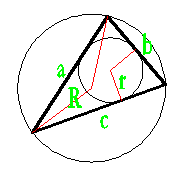## Circumscribed circle of a Triangle calculator

 Side a: Side b: Side c: Inscribed Circle Radius : Inscribed Circle Area : Inscribed Circle Perimeter :

every triangle has a unique circumcircle, a circle passing through all three vertices, whose center is the intersection of the perpendicular bisectors of the triangle's sides.

1. Radius (R) = abc / 4√s(s-a)(s-b)(s-c)
2. s = (a + b + c) / 2Thinkcalculator.com provides you helpful and handy calculator resources.